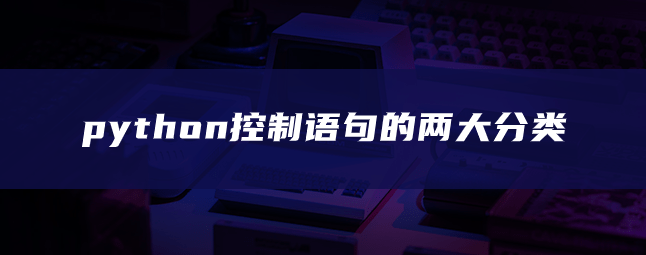## python控制语句的两大分类

244次阅读1、Python为迭代提供了标准的while语句和非常强大的for语句。当给定条件为真时，while语句将重复执行一段代码。

```count=1
while count<=5:
print("河南加油！")
count+=1
#结果如下：

for item in '河南加油！':
print(item)
# 结果如下

！

for i in range(5):
print(i)
# 结果如下
0
1
2
3
4

#通过for语句输出单个字符
wordlist = ['cat','dog','rabbit']
letterlist = []
for aword in wordlist:
for aletter in aword:
letterlist.append(aletter)

letterlist
#结果如下：
['c', 'a', 't', 'd', 'o', 'g', 'r', 'a', 'b', 'b', 'i', 't']```

2、分支语句允许程序员询问，然后根据结果采取不同的行动。

```import math
n=float(input("请输入一个数"))
if n < 0:
print("Sorry,")
else:
print(math.sqrt(n))
#结果：请输入一个数 3
# 1.7320508075688772

#多条件分支
score=float(input("请输入一个数"))
if score >= 90:
print('A')
elif score >= 80:
print('B')
elif score >= 70:
print('C')
elif score >= 60:
print('D')
else:
print('F')
# 请输入一个数 90 A```